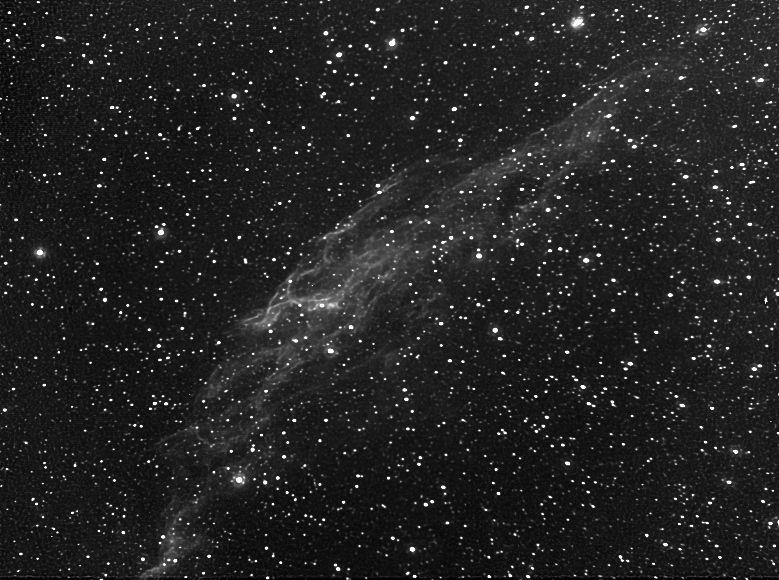Correcting Coma/Field-Curvature Type Aberrations With Deconvolution

Here's a stack of several calibrated images of NGC 6992 (the Veil nebula):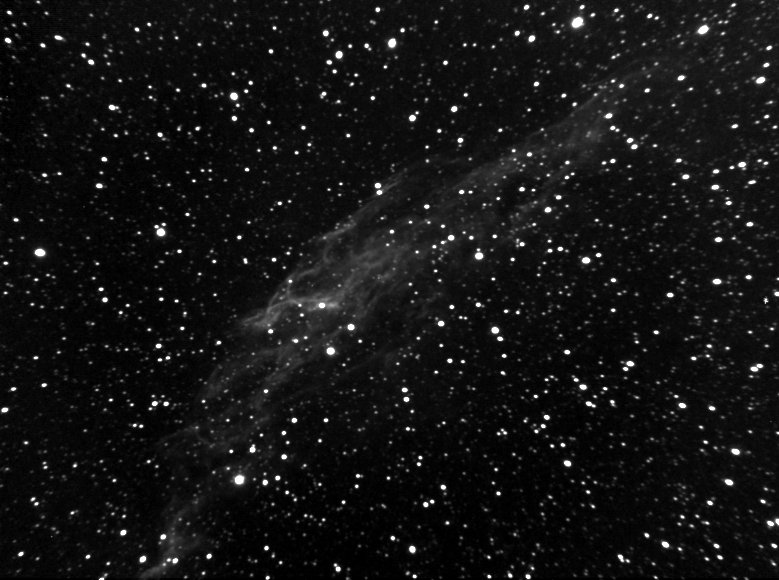The image appears soft because it is slightly out of focus (I think the temperature dropped significantly over the time frame during which the individual exposures were taken). One would like to sharpen the image. Here's the result obtained using the Richardson-Lucy deconvolution algorithm as implemented in Aip4Win: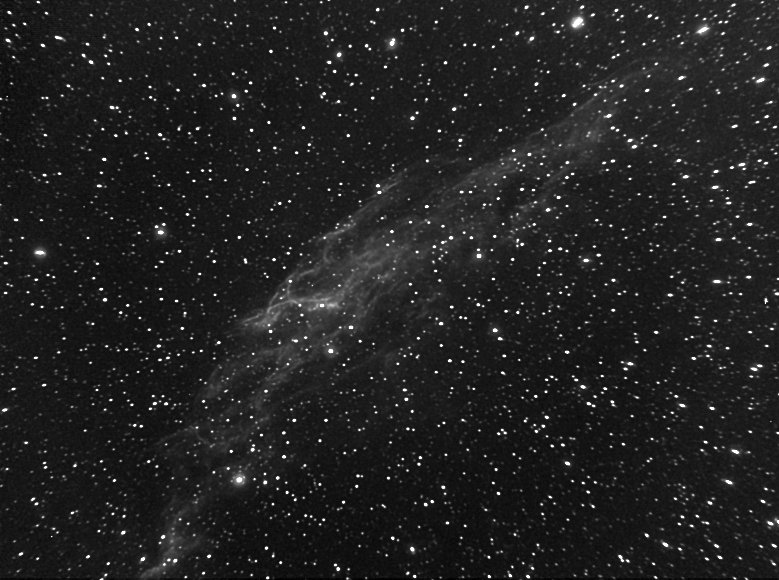Note that the nebulosity in the Veil has improved dramatically, but also note that the stars around the edges now appear seriously distorted. This coma-type distortion appears in all images taken with a focal reducer on my Questar (I've tried three different reducers at several spacings). The question is: can we fix the problem in software.

The Richardson-Lucy deconvolution algorithm became famous when it was applied with dramatic results to the blury images produced by the Hubble Space Telescope when it had its original flawed optics. Maybe it can help us here.

Deconvolution algorithms start with a given point-spread-function (PSF) and attempt to sharpen the image so that copies of the PSF become points of light. We can correct for the coma-type aberration by using a PSF that varies from place-to-place in the image. Unfortunately, none of the commercially available deconvolution packages at my disposal provide this capability. So, I wrote my own. It works as follows. Each PSF is assumed to be Gaussian with a nontrivial covariance matrix. The covariance matrix can be specified by giving the major and minor radii and a corresponding orientation. I assume that the minor radius is constant throughout the image but that the major radius increases with distance from a central point of the image. I also assume that the major axis is oriented to point through this central point. The tool does a quick statistical analysis to guess appropriate values for (a) the minor radius, (b) the major radius at the center of the image, and (c) the major radius at a corner of the image. These initial guesses can be overridden with user-specified values until the PSF's look similar to those in the image.

For NGC 6992, I used the following values for the parameters:

• major radius at center = 1.2
• major radius at corner = 2.5
With these values, the PSFs for 100 bright stars in the raw image are modeled as follows: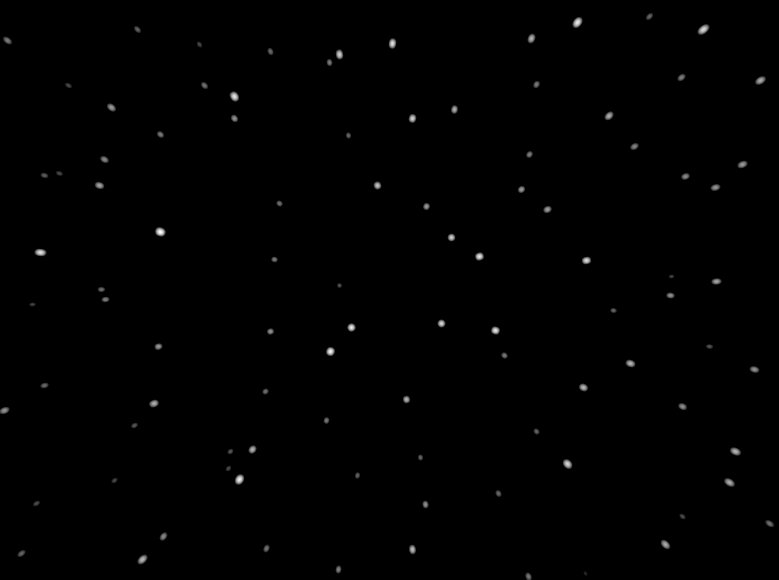I implemented the Richardson-Lucy deconvolution algorithm using this nonconstant model for the PSF. The resulting image after 10 iterations of the algorithm is shown here: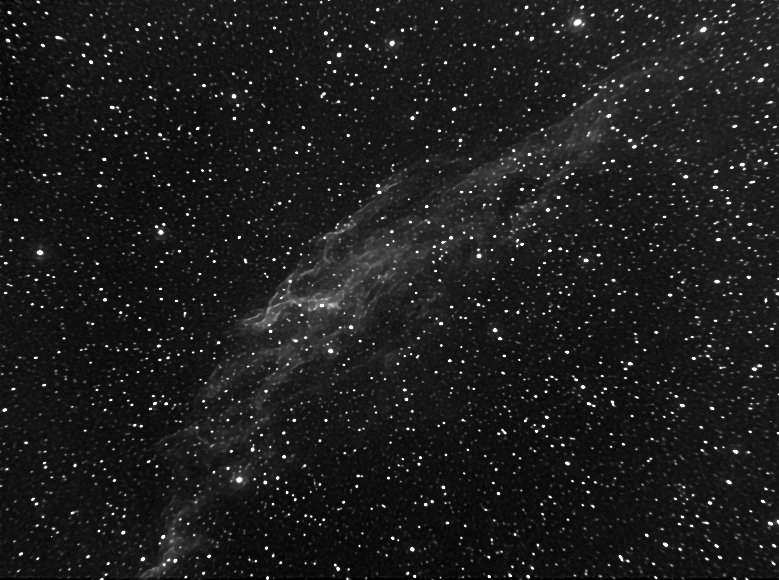More iterations gives even tighter stars but also begins to look overprocessed. Here's the result after 25 iterations: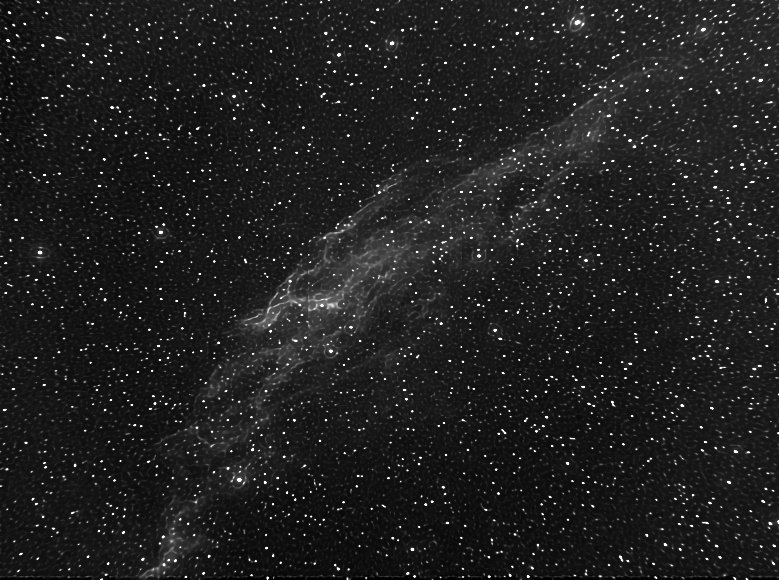A better approach is to break the problem into two parts: (a) first, make the stars round, and then (b) make the image sharper. The "rounding" step can be done, for example, by doing 10 iterations with